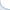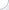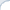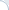## Getting up to speed with neural machine translation : How not to burn yourself with PyTorch

Last week I shared my time between work and hacking at a forth machine translation marathon in the Americas. This event organized jointly by CMU and Amazon (sponsored by Amazon) was a lot of fun. My small sub-team of two people got familiar with OpenNMT, trained English-German and English-Ukrainian models, as well as implemented an idea of our team lead Adithya Renduchintala. Hey, we have even gotten a tiny 0.5 gain in BLEU for the English-Ukrainian pair!

We certainly learned a lot of lessons, most of which generalize well beyond the neural machine translation task. One is related to implementation of custom neural modules and PyTorch. Unlike TensorFlow and many other packages, PyTorch belongs to a new crop of neural frameworks, where a neural network (computation) graph is dynamic. What does it mean? It means that you do not have define a computation graph in advance. You can simply write a tensor-manipulating code and PyTorch will do all the back-propagation and parameter updating automatically. Another well-known package with a similar functionality is DyNet.

There are ups and downs to dynamic computation graphs. For one thing, it is much simpler to debug them. For another, there is a lot of magic going behind the scenes, which you need to understand. First of all, one needs to remember that the computation graph is defined by a sequence of manipulations on Tensors and Variables (Variable is a Tensor wrapper that got deprecated in the recent PyTorch). Your sequences should be valid and properly linked so that all the Tensors of interest have a chance to be updated during back-propagation.

Tensor-level manipulations can easily get hairy. To simplify things, PyTorch introduces an abstraction layer called Module. A Module is a basic building block that has some parameters and a function forward to turn inputs to outputs. If all is done properly, given only the forward function PyTorch can compute the gradients via back-propagation and update the model parameters. The nice thing about PyTorch is that you can easily write a new module by combining several existing ones. There is no need to write arcane description of layers! As we can see from this PyTorch example, we can define a forward network computation in a very straightforward way. Even if you have not seen a line of PyTorch code before, you can easily figure out that this module applies two 2d-convolutions each of which is followed by a RELU non-linearity:

class Model(nn.Module):    def __init__(self):        super(Model, self).__init__()        self.conv1 = nn.Conv2d(1, 20, 5)        self.conv2 = nn.Conv2d(20, 20, 5)     def forward(self, x):        x = F.relu(self.conv1(x))        return F.relu(self.conv2(x))

But here is a catch that is barely mentioned in PyTorch documentation. Although writing the forward function is sufficient to compute the gradients, it is apparently not sufficient to determine which tensors represent module's parameters. In the above example, the module includes two convolutional neural networks, each of which has parameters to be updated. How does PyTorch know this? Well, turns out that PyTorch overloads the function __setattr__! Thus, it surreptitiously "registers" each submodule when a user makes an assignment like this one:

self.conv1 = nn.Conv2d(1, 20, 5)

Unfortunately, such automatic registration does not work all the time. Imagine, for example, you want to aggregate several sub-modules whose number is not known in advance. It is very natural to save them all in a list:

class Model(nn.Module):    def __init__(self):        super(Model, self).__init__()        self.sub_modules = []        self.sub_modules.append(nn.Conv2d(1, 20, 5))  # append a module to the list        self.sub_modules.append(nn.Conv2d(20, 20, 5)) # append a module to the list

Yet, this is where PyTorch magic stops: If you place the modules in plain Python list, PyTorch will not be able to update their parameters. As a fix, you need to register them explicitly. One way to do this is to explicitly call the function add_module. A less tedious ways is to use a combination of nn.ModuleList and nn.Sequential. Please, read a discussion here for more details.

## Efficient and Accurate Non-Metric k-NN Search with Applications to Text Matching: We Need More k-NN Search!

The final version of my thesis "Efficient and Accurate Non-Metric k-NN Search with Applications to Text Matching" is now available online. An important by-product of my research is an efficient NMSLIB library, which I develop jointly with other folks. In a podcast with Radim Řehůřek (Gensim author) I discuss this project, its goals, and its history in detail.

Although efficiency is an important part of the thesis, it is primarily not about efficiency. Most importantly, I try to deliver the following messages:

1. We have very flexible retrieval tools, in particular, graph-based retrieval algorithms, which can work well for a wide variety of similarity functions. In other words, we do not have to limit ourselves neither to inner-product similarities (e.g., the Euclidean distance) nor to even metric spaces.
2. When "queries" are long, these algorithms can challenge traditional term-based inverted files. So, in the future, I expect retrieval systems to rely less on classic term-based inverted files and more on generic k-NN search algorithms (including graph-based retrieval algorithms). I think it is not a question of "IF", but rather a question of "WHEN".

Graph-based retrieval is an old new idea, which has been around for more than twenty years. This idea is beautifully simple: Build a graph where sufficiently close points are connected by edges. Such graphs come in various flavors and can be collectively called neighborhood graphs or proximity graphs. Given a neighborhood graph, nearest neighbor and other queries can be answered (mostly only approximately) by traversing the graph in a direction towards the query (and starting from, e.g., a random node). I cover the history of this idea in my thesis in more detail, but the earliest reference for this approach that I know is the seminal paper by Sunil Arya and David Mount (BTW, David Mount is co-author of the well-known ANN library).

Despite this early discovery, the practicality of graph-based retrieval in high-dimensional spaces was limited because we did not know how to construct neighborhood graphs efficiently. As it often happens in science, a number of fancy methods were proposed (while overlooking a simpler working one). Luckily, it was discovered that the graph can be constructed by iteratively building the graph and using a graph-based retrieval algorithm to find nearest neighbors for a new data point. A summit (or at least a local maximum) of this endevour is a Hierarchical Navigable Small World graph (HNSW) method, which combines efficient pruning graph-pruning heuristics, a multi-layer and multi-resolution graph topology with a bunch of efficiency tricks.

It was also known (but not well-known) that graph-based retrieval algorithms can work for generic (mostly metric) distances. So, I personally was interested in pushing these (and other) methods even further and applying them to non-metric and non-symmetric similarities. One ultimate objective was to replace or complement a standard term-based inverted file in the text retrieval scenario. Well, the idea to apply k-NN search to text retrieval is not novel (see, again, my thesis for some references). However, I do no think that anybody has shown convincingly that this is a viable approach.

On the way towards achieving this objective, there are a lot of difficulties. First of all, it is not clear which representations of text and queries one can use (I have somewhat explored this direction, but the problem is clearly quite hard). Ideally, we would represent everything as dense vectors, but I do not think that the cosine similarity between dense vectors is particularly effective in the domain of adhoc text retrieval (it works better for classification, though). I am also convinced that in many cases whenever dense representations work well, a combination of dense and sparse bag-of-words representations works even better. Should we embrace these hybrid representations in the future, we cannot use traditional term-based inverted files directly (i.e., without doing a simpler search with subsequent re-ranking). Instead, we are likely to rely on more generic algorithms for k-nearest neighbor (k-NN) search.

Second, instead of trying to search using a complex similarity, we can use such a similarity only for re-ranking. Of course, there should be obviously limits to the re-ranking approach. However, a re-ranking bag-of-words pipeline (possibly with some query rewriting) is a baseline that is hard to beat.

Third, k-NN search is a notoriously hard problem, which in many cases cannot be solved exactly without sequentially comparing the query with every data point (the so called brute-force search). This is due to a well-known phenomenon called the curse of dimensionality. Often we have to resort to using approximate search algorithms, but these algorithms are not necessarily accurate. How much inaccuracy is ok? From my experimental results I conclude that the leeway is quite small: We can trade a bit of accuracy for extra efficiency, but not too much.

Because approximate k-NN search leads to loss in accuracy, in my opinion, it does not make sense to use it with simple similarities like BM25. Instead, we should be trying to construct a similarity that beats BM25 by a good margin and do retrieval using this fancier similarity. My conjecture is that by doing so we can be more accurate and more efficient at the same time! This is one of the central ideas of my thesis. On one collection I got promising results supporting this conjecture (which is BTW an improvement of our CIKM'16 results). However, more needs to be done, in particular, by comparing against potentially stronger baselines.

In conclusion, I note that this work would have been impossible without encouragement, inspiration, help, and advice of many people. Foremost, I would like to thank my advisor Eric Nyberg for his guidance, encouragement, patience, and assistance. I greatly appreciate his participation in writing a grant proposal to fund my research topic. I also thank my thesis committee: Jamie Callan, James Allan, Alex Hauptmann, and Shane Culpepper for their feedback.

I express deep and sincere gratitude to my family. I am especially thankful to my wife Anna, who made this adventure possible, and to my mother Valentina who encouraged and supported both me and Anna.

I thank my co-authors Bileg Naidan, David Novak, and Yury Malkov each of whom greatly helped me. Bileg sparked my interest in non-metric search methods and laid the foundation of our NMSLIB library. Yury made key improvements to the graph-based search algorithms. David greatly improved performance of pivot-based search algorithms, which allowed us to obtain first strong results for text retrieval.

I thank Chris Dyer for the discussion of IBM Model 1; Nikita Avrelin and Alexander Ponomarenko for implementing the first version of SW-graph in NMSLIB; Yubin Kim and Hamed Zamani for the discussion of pseudo-relevance feedback techniques (Hamed also greatly helped with Galago); Chenyan Xiong for the helpful discussion on embeddings and entities; Daniel Lemire for providing the implementation of the SIMD intersection algorithm; Lawrence Cayton for providing the data sets, the bbtree code, and answering our questions; Christian Beecks for answering questions regarding the Signature Quadratic Form Distance; Giuseppe Amato and Eric S. Tellez for help with data sets; Lu Jiang for the helpful discussion of image retrieval algorithms; Vladimir Pestov for the discussion on the curse of dimensionality; Mike Denkowski for the references on BLUE-style metrics; Karina Figueroa Mora for proposing experiments with the metric VP-tree applied directly to non-metric data. I also thank Stacey Young, Jennifer Lucas, and Kelly Widmaier for their help.

I also greatly appreciate the support from the National Science Foundation, which has been funding this project for two years.

## On adversarial examples and space-partitioning capabilities of neural networks

A tree-based machine learning method splits the space into regions and fits a region-specific classification/regression function (typically just a constant). Additional flexibility and capacity can be achieved by bagging and boosting elementary trees, but at the core of these methods is their space partitioning capabilities. A few months ago I started to wonder if neural networks can do a similar thing. My gut feeling was that neural nets should be doing something of the kind, but the mechanics of the neural net space partitioning was unclear to me. I even posted a question on StackExchange, yet, I have not gotten an answer.

However, I recently discovered an insightful video lecture by Ian Goodfellow where he elaborates on the nature of adversarial examples and discusses possible ways to defend from an attacker (spoiler alert: there seems to be no working method yet!). Basically, he argues that adversarial examples is a common Achilles' heel, which comes—in fact somewhat surprisingly—from excessive linearity of existing approaches. Ian Goodfellow argues that this issue plagues almost all standard machine learning approaches including SVMs, tree-based methods, and neural networks. What is even more surprising, adversarial examples are transferable across different algorithms: An adversarial example designed to fool, e.g., a decision tree is very likely to fool some other method as well!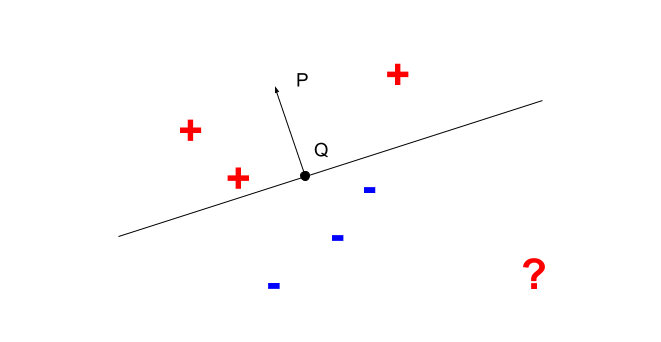#### Fig 1. A hyperplane separating positive and negative examples.

Ian also notes that neural nets divide the space using an approximately piecewise linear function. This observation basically answers my question about the space partitioning capabilities of neural networks. For neural nets with only truly piecewise linear activation functions (such as RELU), the division of the space is truly linear. As a side comment, this kinda explains (at least for me) the ability of neural networks to generalize quite well despite having so many parameters. A well-known deficiency of the linear and piecewise linear models (as noted by Ian) is that they can be super confident in regions where they encountered no training data. For example, in Figure 1 the linear classifier would have a very strong "opinion" about the negativity of the point marked by the red question mark. However, this confidence is not fully substantiated, because there are no training points nearby.

Adversarial examples notwithstanding, I think it may be useful to get a better understanding of the space partitioning "mechanics" of the neural networks. In my case, such understanding came from reading a very recent paper AN UPPER-BOUND ON THE REQUIRED SIZE OF A NEURAL NETWORK CLASSIFIER by Hossein Valavi and Peter J. Ramadge. The paper is unfortunately paywalled, but the slides are publicly available.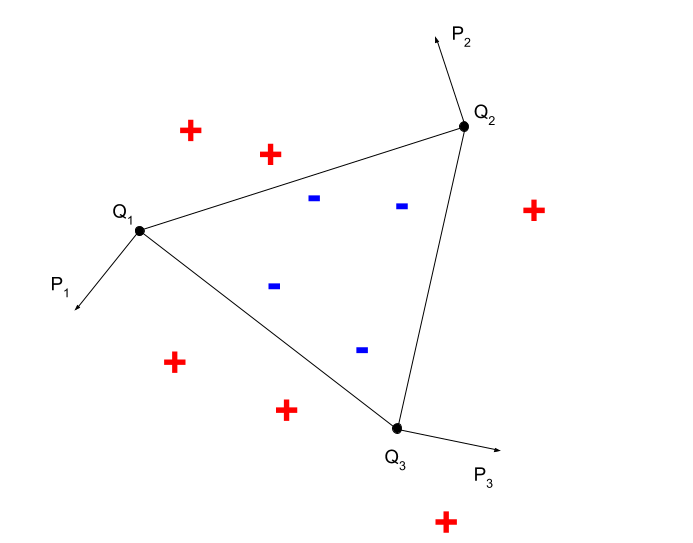#### Fig 2. A convex shape (triangle) classifier.

In this paper, authors remind us that neural nets possess two powerful capabilities: an ability to distinguish data points separated by a given hyperplane and a capacity to implement arbitrary logic circuits (they also prove facts about complexity of such circuits, but these proofs are not very relevant to our discussion). Without going into technicalities right away (but some are nevertheless discussed below), this means that neural nets can easily divide a real-vector space using polytopes, e.g., convex ones. Let us consider a simple example of a piecewise linear classifier in Fig. 2. As we can see positive and negative training examples can be separated from each other using three planar hyperplanes, simply speaking by three lines. Positive and negative examples are located on the opposite sides of these separating lines. Therefore, a condition of belonging to a negative class can be written as : $\langle x - Q_1, P_1 - Q_1 \rangle < 0 \mbox{ and } \langle x - Q_2, P_2 - Q_2 \rangle < 0 \mbox{ and } \langle x - Q_3, P_3 - Q_3 \rangle < 0$. This condition can be easily implemented with a two layer neural networks with the sign activation function.

Because neural nets can have tons of parameters, they can slice and dice the space at least as well as tree-based learning algorithms. In fact, if necessary, given enough parameters, we can always achieve a perfect separation of data points from different classes (assuming consistent labeling). For example, we can place each positive-class datapoint into its own hyperhube and implement neural nets that "fire" only for training data points in these hypercubes. We will then combine these nets using an OR circuit. Of course, such construct is extremely inefficient and does not generalize well, but it IMHO illustrates the basic space-partitioning powers of the neural nets.

In conclusion, I discuss some technical details, which are primarily of the theoretical interest. For simplicity of exposition I consider only the binary classification scenario. First, let us consider again a hyperplane that divides the space into two subspaces. The claim is that we can always construct a neural network that maps points on one side of the hyperplane to zeros (negative) and points on the other side of the hyperplane to ones (positive). This fact is trivial for neural networks with the sign activation function (a sign of the scalar product between data points and the hyperplane-orthogonal vector defines the class of a data point). We illustrate this point in Figure 1. However, it is more work to prove for differentiable activation functions such as RELU (which are actually used in practice nowadays). This is done in Lemma 3.1 of the mentioned paper.

Once we divide the space into regions and implement all neural networks that fire exactly when a data point is in its region, we need to combine these classifiers using a boolean circuit. Consider again an example in Fig. 2 In this case a boolean circuit is a simple three-variable conjunction.

There are certainly many ways to implement a binary logic circuit using a neural network. One of these is briefly outlined Corollary 3.3. This Corollary is a bit hard to parse, but, apparently, authors propose to solve a problem in two steps. First, they suggest to map each combination of $n$ zeros and ones to a single-coordinate data point in a $2^n$-dimensional space. Because circuit values can be true or false, there will be "true" or "false" data points in the target $2^n$-dimensional space. If coordinate values of true and false points are different, these points are linearly separable (e.g., a "true" data point has exactly one dimension with value one and a "false" data point has exactly one dimension with value two). Thus, according to their Lemma 3.1, there would exist a second neural network based function that maps true points to one and false points to zeros.

Authors do not describe how to implement the mapping to the $2^n$ dimensional space, but we can use the following combination of RELU-activated threshold circuits:

$\max(0, \sum 2^i \cdot x_i - k + 1) - 2\max(0, \sum 2^i \cdot x_i - k) + \max(0, \sum 2^i \cdot x_i - k - 1)$.

Given a binary input variables $x_i$ this function is equal to one if and only if the input is the same as the binary representation of $k$.

UPDATE: quite interestingly a recent paper provides a more specific characterization of functions represented by feed-forward neural networks with RELU activations. Namely, this is a subclass of rational functions belonging to the family of tropical rational maps (a tropical map is piecewise linear).

## NLP approaches/tools to automatically rewrite sentences

I was asked on Quora about NLP approaches or tools to automatically rewrite sentences. Here is my brief answer (feel free to vote on Quora).

One of the following approaches can be used:

1) Manual rules. These can be either regexp based or tree-based. Stanford folks have a tool to create such patterns. One interesting option is to implement rules based on POS tags. I, e.g., reimplemented some of the query-rewriting algorithms from Aranea QA system. This is not easy and the quality is sometimes not ideal.

2) Learned from data. One common approach to do this is, perhaps, surprisingly machine translation. This would require a rather large monolingual corpus of paired sentences. One sentence would be source and another would be a target. You can train all sorts of translation models on such a corpus starting from simplistic Model 1 and ending with some phrase-based model (or even context-free grammar based, see the link below).

3) It is also possible to obtain paraphrasing information by pivoting on a foreign language. Here is one link PPDB: The Paraphrase Database. You may want to read papers authored by the guys who created PPDB (you can use machine translation directly for this as explained by Vasily Konovalov)

4) A more recent approach relies on neural sequence-to-sequence (seq2seq) models. One recent paper on this subject is: Neural Paraphrase Generation with Stacked Residual LSTM Networks, Prakash et al., 2016.

## Pages